Massachusetts is moving to the new national standards (Common Core) and related tests (PARCC). At least so it seems. The politics are complex and hard to predict, and they’ll play out over the next few years.

This post is not about the politics, though. It’s about the substance of it all—how the new standards and tests look here on the ground to our students and teachers.

This post is the first of two. It addresses the move from the Bay State’s MCAS exams to PARCC in math.

A second post, later this week, will cover the move from MCAS to PARCC in ELA.

I prefer PARCC-Math over its predecessor, the MCAS-Math. Here are some of the reasons why.

1.) PARCC-Math mitigates the role of luck.

Guessing is invariably a part of test taking, but less so with PARCC-Math than with MCAS-Math.

A typical MCAS-Math multiple-choice question asks a student to select one of four correct answers. For example, consider this order-of-operations question from the fifth-grade MCAS-Math:A student with no knowledge of order of operations has a 25 percent chance of guessing the correct answer.

PARCC-Math greatly reduces a student’s odds of lucking into the correct answer. PARCC-Math asks fewer straight multiple choice questions, instead favoring open-response questions. Where PARCC-Math seeks a simple numerical answer, it often asks students to fill out an answer grid rather than offering a series of possible answers.

Here is an example of an order-of-operations question from fifth-grade PARCC-Math, one that involves a “grid” answer key: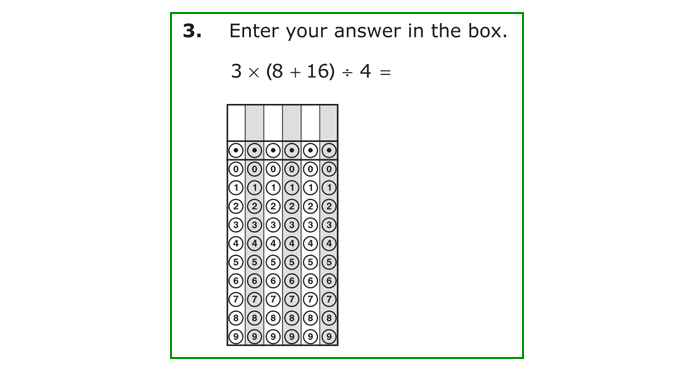Even when PARCC does ask standard multiple choice questions, it often asks the student to select “each” correct answer without specifying how many correct answers are present in the answer lineup.

Here is an example of a seventh-grade PARCC-Math multiple choice question with multiple correct answers: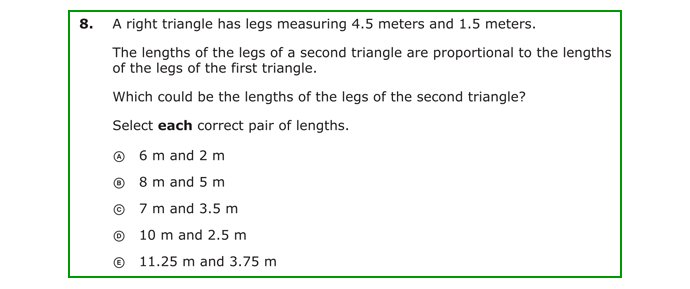The question above has two correct answers (A and E). The odds of randomly guessing A and E are miniscule (about 3 percent). Students cannot easily luck into showing mastery on PARCC-Math.

2. PARCC-Math, more than MCAS-Math, requires students to understand math conceptually.

Consider the following seventh grade MCAS-Math question on volume: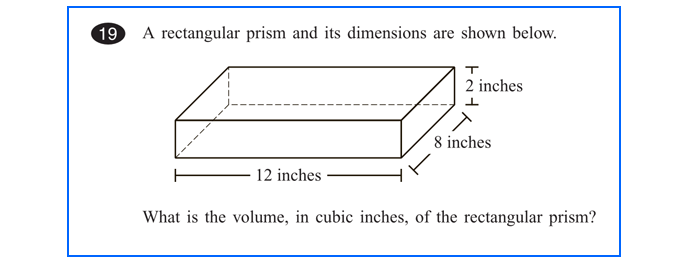To answer this question, a student needs simply to apply the formula for volume (length x width x height) but does not need to understand volume conceptually (i.e., be able to spot a volume question that is not overtly described as such).

Moreover, in the question above, the values for the formula are obvious. A student does not need to be selective in identifying relevant data to input into the volume formula.

For contrast, consider this seventh-grade PARCC-Math question on volume: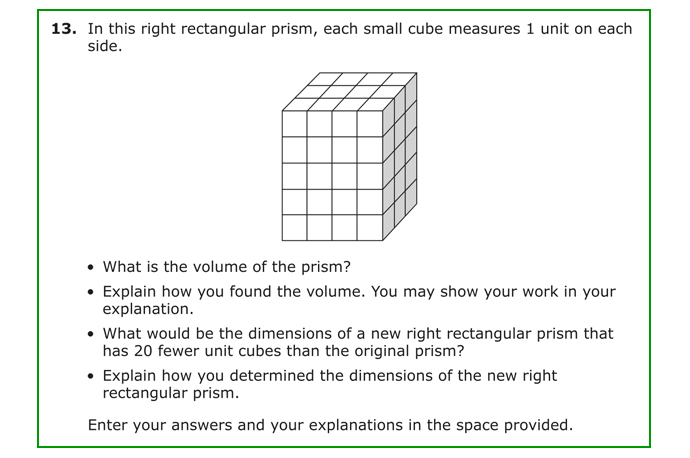In this PARCC-Math volume question, a student still needs to know and be able to apply the basic formula for volume of a rectangular prism. But notice the additional challenges involved in the PARCC-Math version.

First, students have to arrive independently at the values for length, width, and height in the volume formula. They are not given or obvious. That challenge alone will stump students who have only a superficial understanding of volume.

Second, students have to know the meaning of a “right rectangular prism” in order to downsize the block correctly by twenty units, as directed in the third bullet of the question.

And third, students have to show their work and logic as they progress through the problem.

In short, students truly have to understand volume as a phenomenon in order to answer this question. Mastering the formula for volume alone is not enough.

3. PARCC-Math, more than MCAS-Math, requires students to provide detailed explanations for their solutions.

Historically, MCAS-Math rarely asked students to explain their choice of math algorithm. For example, consider this fifth-grade MCAS-Math problem on fractions: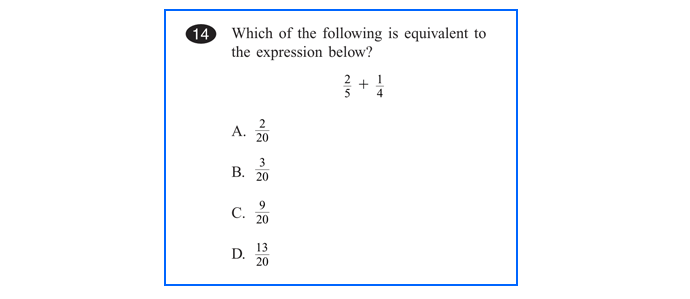By comparison, consider this fifth-grade PARCC-Math question, also on the addition and subtraction of fractions: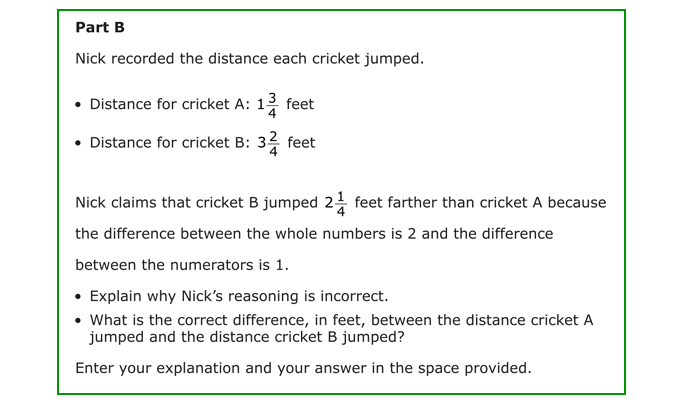To get full credit on this PARCC-Math question, a student obviously needs to be able to subtract 1 3/4 from 3 2/4

But she also needs to understand this procedure as the one that is called for in the word problem and—for full credit—to go further and explain how the protagonist mishandled the problem.

The question, far more than a conventional MCAS-Math question, demands fluency with fractions.

4. PARCC-Math open response questions, more than their MCAS analogs, demand that students identify relevant information and solve complex, multi-step problems.

The most challenging MCAS-Math questions are “open-response” questions. These questions typically ask a string of two or three questions, sequenced in a way that guides a student through a problem.

Here is an example of an MCAS-Math open-response question in fifth grade. It deals mainly with multiplication and division: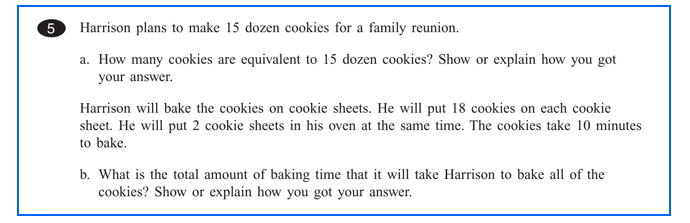This question is, in our opinion, a solid test of a student’s mastery of multiplication and division. But it could be a lot more demanding.

In particular, notice how a question is inserted immediately following the information relevant to that question. In this way, the question guides students.

By contrast, PARCC-Math open-response questions tend to present a full and often lengthy word problem and then, at the end, ask a series of questions that require students to sort for and manipulate relevant information.

Here is a fifth-grade PARCC-Math open-response question that also tests multiplication and division. It is much harder, as you will see: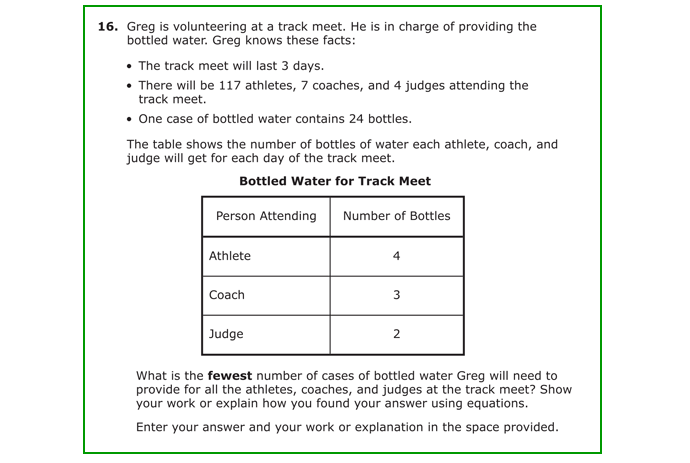On this PARCC-Math question, students have to take seven or eight steps to reach a solution, whereas the MCAS-Math analog above required only three or four. And students have to search intensively for information relevant to each step of the solution. The question truly tests students’ ability to parse information in context, to think conceptually, and to discern an efficient path to a solution.

This question also involves numbers that are plainly more difficult to multiply and divide than the numbers on the MCAS-Math questions. The last step on the PARCC-Math question involves dividing 1,491 by 24, finding the answer as 62 with remainder 3, and concluding as a result that at least 63 cases of water are needed. By comparison, the most complex operation on the MCAS-Math question is to divide 180 by 18.

Conclusion

The new tests and the Common Core standards offer a more rigorous approach to math skills and knowledge than their predecessors, in my view. They prepare our students more clearly and from an earlier age for the challenges of advanced math (algebra, geometry, trigonometry, and calculus) that they will face at our high school and college.

PARCC-Math is a challenge worthy of our students and teachers.

– Stig Leschly

Stig Leschly is the CEO of Match Education.

This post originally appeared on the Fordham Institute’s Common Core Watch. Before that it appeared in a slightly different form at Puzzl-ED.

Last updated July 8, 2015

July 7, 2015

July 9, 2015